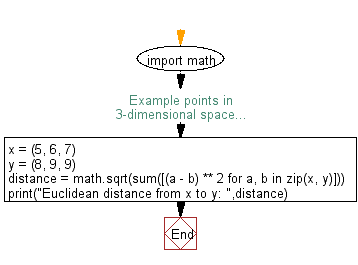﻿ Python Math: Compute Euclidean distance - w3resource# Python Math: Compute Euclidean distance

## Python Math: Exercise-79 with Solution

Write a Python program to compute Euclidean distance.

Note: In mathematics, the Euclidean distance or Euclidean metric is the "ordinary" (i.e. straight-line) distance between two points in Euclidean space. With this distance, Euclidean space becomes a metric space. The associated norm is called the Euclidean norm.

Sample Solution:-

Python Code:

``````import math
# Example points in 3-dimensional space...
x = (5, 6, 7)
y = (8, 9, 9)
distance = math.sqrt(sum([(a - b) ** 2 for a, b in zip(x, y)]))
print("Euclidean distance from x to y: ",distance)
```
```

Sample Output:

```Euclidean distance from x to y:  4.69041575982343
```

Flowchart:## Visualize Python code execution:

The following tool visualize what the computer is doing step-by-step as it executes the said program:

Python Code Editor:

Have another way to solve this solution? Contribute your code (and comments) through Disqus.

What is the difficulty level of this exercise?

Test your Programming skills with w3resource's quiz.

﻿

```>>> students = [{'name': 'John', 'score': 98}, {'name': 'Mike', 'score': 94}, {'name': 'Jennifer', 'score': 99}]